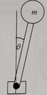# Tension and reaction force in circular motion

• Like Tony Stark
In summary, when the rod is in compression, there is a negative force and when the rod is in tension, there is a positive force.f

#### Like Tony Stark

Homework Statement
Consider a rotating rod with a mass attached to its end.
When does the rod produce a compression force and when a tension force?
Relevant Equations
##\hat n) F_n=mr\omega^2##
Hi
I'm having trouble to understand the centripetal force in a rotating rod with a mass in its end.
When ##90°<\theta<270°##, the centripetal acceleration is produced by the tension, which counteracts the radial component of the weight.
But what happens when ##\theta<90°## or ##\theta>270°##? There's no tension because the force (weight) is towards the centre, so the weight causes the centripetal acceleration. Right?
As the rod is rigid, I think that, for some interval of ##\theta##, the rod produces a force that acts as a tension force and for other interval it acts as a compression force. Would this interval be compression for ##\theta<90°## and tension for ##90°<\theta<270°##?
Then, in the case of a string instead of the rod, the centripetal acceleration is produced by the tension and when ##\theta<90°## it is produced by the weight.

#### Attachments

•20200912_180411.jpg
3.4 KB · Views: 68
you did not consider gravity?
what if ##\omega## is really large, like ##\ \gt \sqrt{g/r\ }## ?

Hi. There are some misconceptions in what you have written. See if this helps. Sorry it's rather long.

Sometimes, extreme cases give a physical insight. Suppose the mass is moving really fast. Intuitively, you should ‘get’ that the rod is always in tension (like a string would be). So your statement:
“There's no tension because the force (weight) is towards the centre,”
is wrong in this situation.

I probably shouldn’t explain in this detail, but see if it helps...

For convenience, use the convention: radially inwards is positive, and radially outwards is negative. So if the rod is in tension, T>0, as the rod is pulling the mass radially inwards. And, correspondingly, if the rod is in compression, we can say T<0.

For a mass M on the end of a rod (length L), rotating at constant speed in a vertical circle, the resultant force, F, acting on M is the vector sum of:
1) the radial component of weight, m|g|cosθ;
2) the tension or compression in the rod, T.
(I’ve used |g| to avoid confusion if taking g as a negative value).

If M is in the upper half of the circle, cosθ is positive so the radial component of weight (m|g|cosθ) is positive, acting radially inwards, as it must.

If M is in the lower half of the circle, cosθ is negative so the radial component of weight (m|g|cosθ) is negative, acting radially outwards, as it must.

And note that T is not a constant force. T is a function of M, L, speed(v) and θ. T continuously changes throughout each revolution.

If M, L and v are constant we can treat T as a function of θ, so write it as T(θ)

I’ll explain how we can work out T(θ) for any value of θ, and why it can be positive or negative.

Resultant force on M is:
F = T(θ) + m|g|cosθ

For circular motion at constant speed v, centripetal force = resultant force = mv²/L so:
mv²/L = T(θ) + m|g|cosθ
T(θ) = mv²/L - m|g|cosθ
= m(v²/L - |g|cosθ)

If v²/L > |g|cosθ then T is positive so rod is in tension.
If v²/L <|g|cosθ then T is negative so rod is in compression.

If you think about it, this shows for large enough values of v, T is always in tension.
And for small values of v, T is in compression for part of the rotation and in tension for the other part.

(Sorry, for speed I didn't use Latex.)

•Like Tony Stark